# NCERT Exemplar Solutions for Class 9 Maths Chapter 7 - Triangles

## NCERT Exemplar Solutions Class 9 Maths Chapter 7 – Free PDF Download

NCERT Exemplar Class 9 Maths Chapter 7 Triangles, is provided here for students to prepare for exams and score good marks. These NCERT Exemplar Class 9 Maths problems have been designed in accordance with CBSE Syllabus (2021-2022) for 9th standard by our experts, which covers the following topics of chapter Triangles given below:

• Congruent triangles
• Criteria’s or conditions for congruency of triangles
• Inequalities in a Triangle

Triangles are one of the most interesting and easy chapters of Class 9 Mathematics. In this chapter, the students will learn the basic concepts of triangles like congruence of triangles, criteria for congruence of triangles like Side Angle Side, Angle Side Angle, Angle Angle Side. To get these concepts understood we are providing here exemplar solutions, which students can also use as a reference tool while solving NCERT Book exercise questions.

## Download PDF of NCERT Exemplar Solutions for Class 9 Maths Chapter 7 Triangles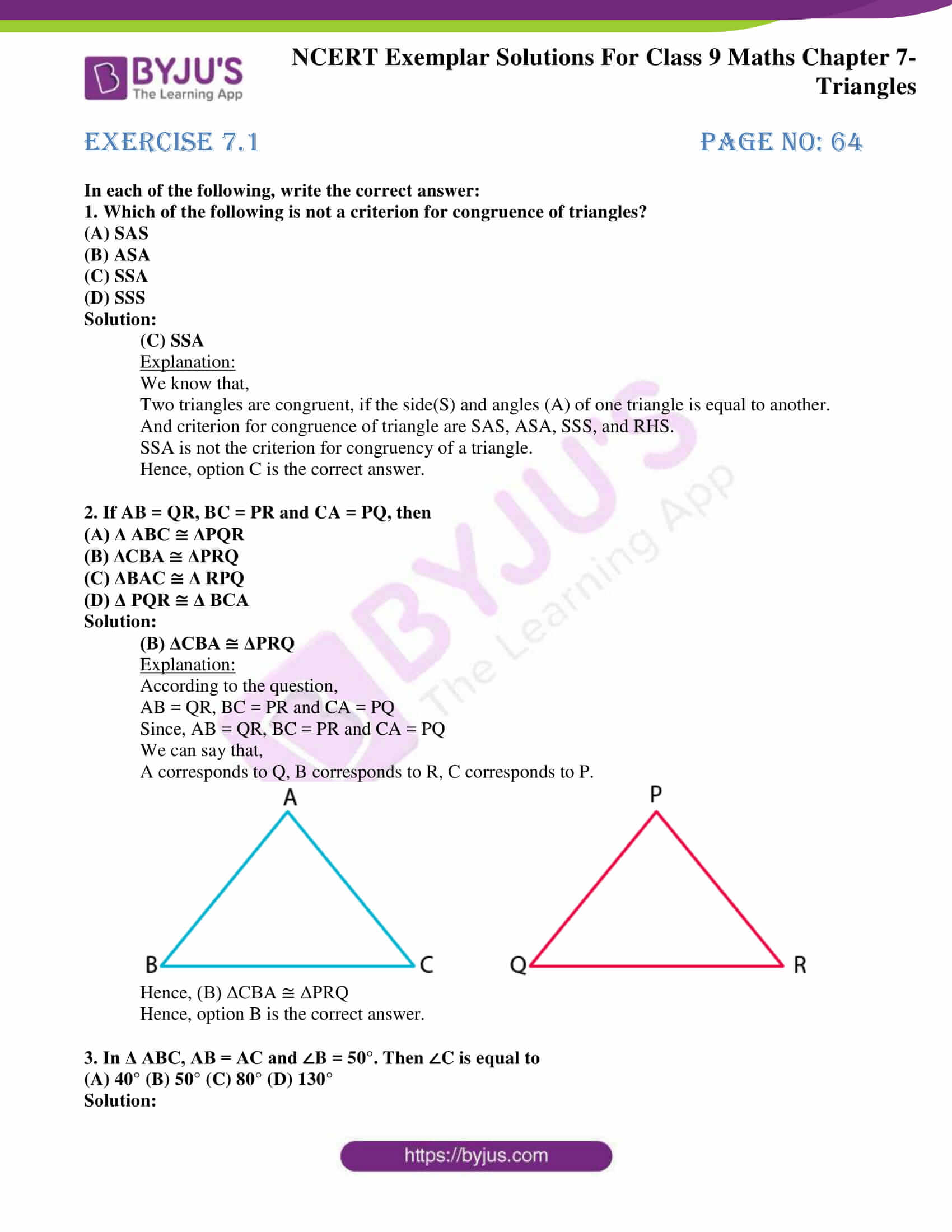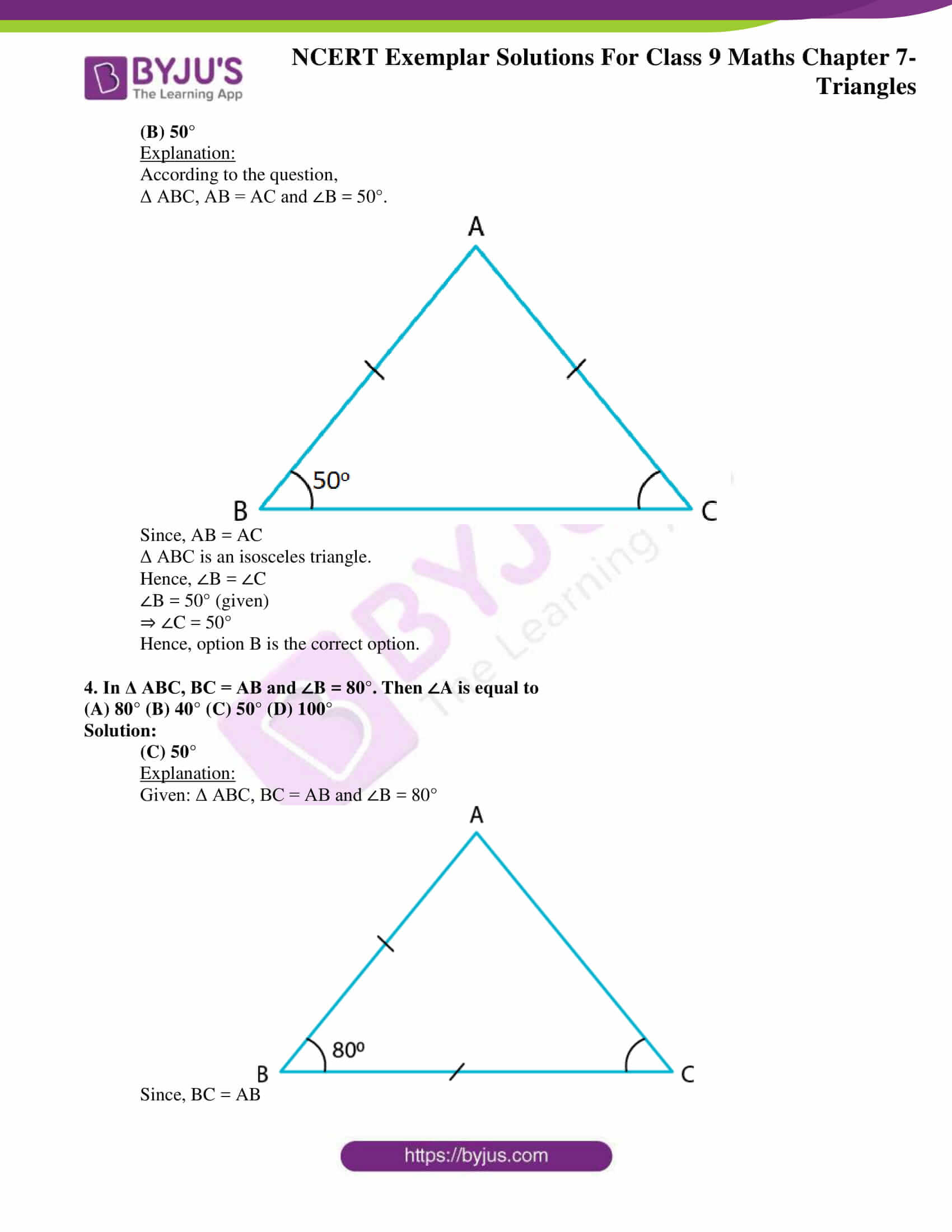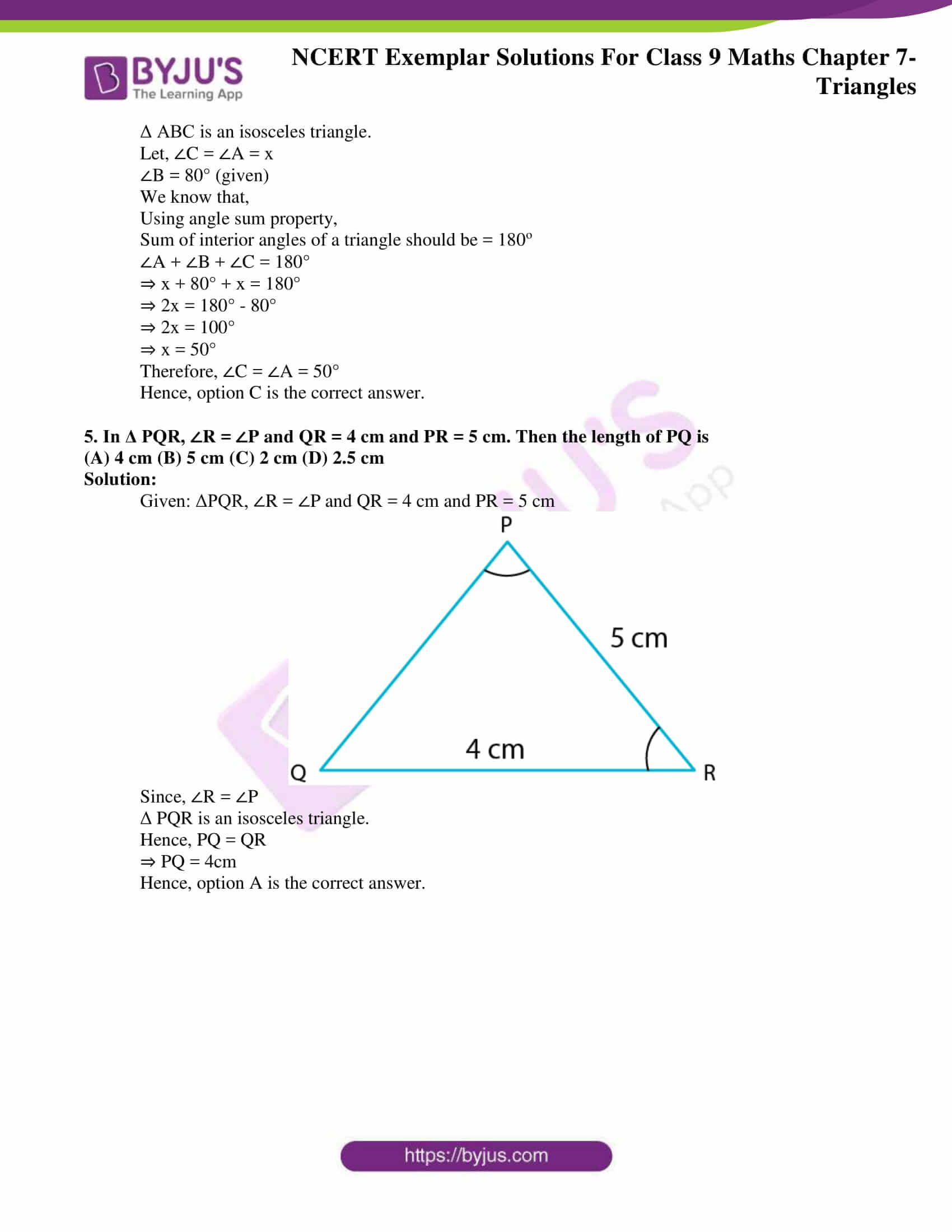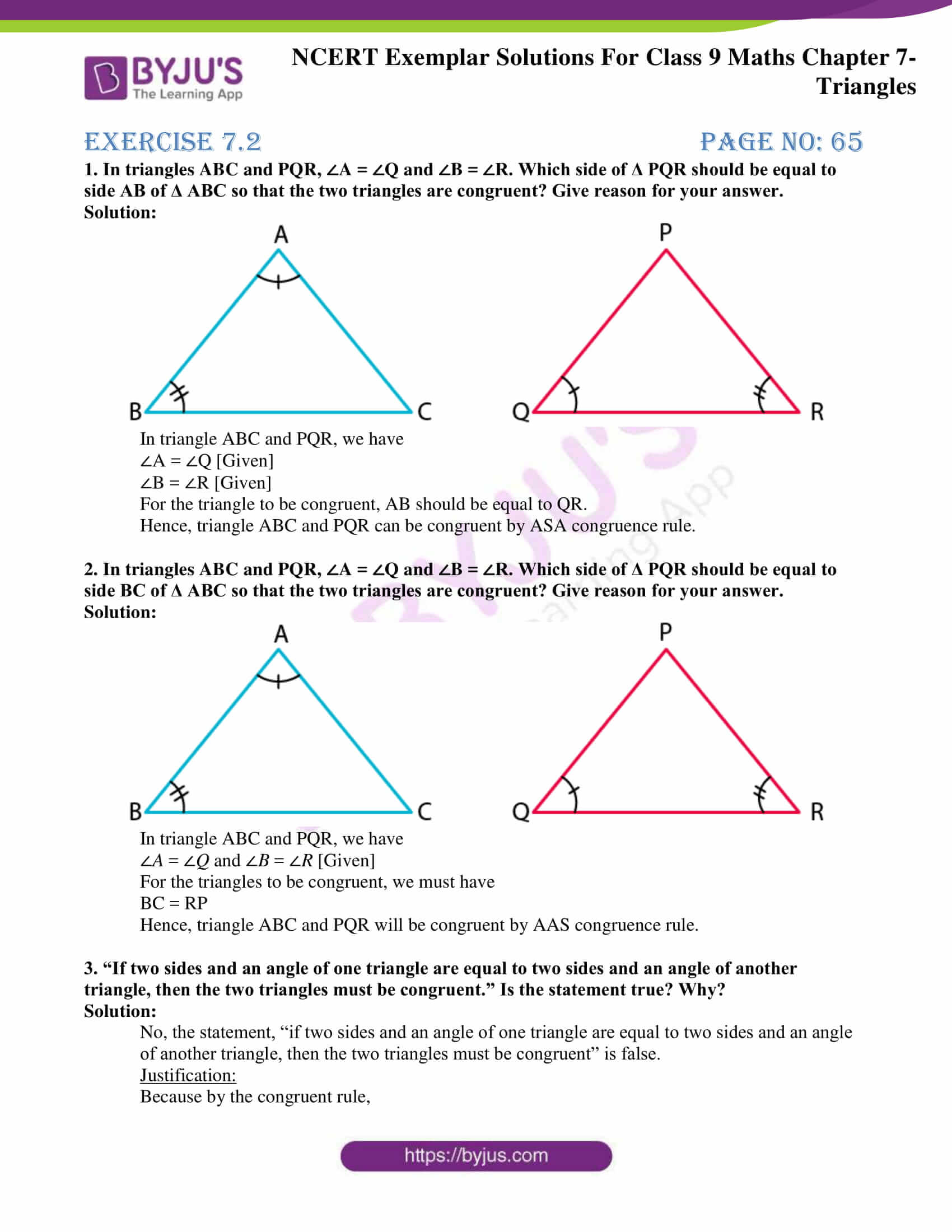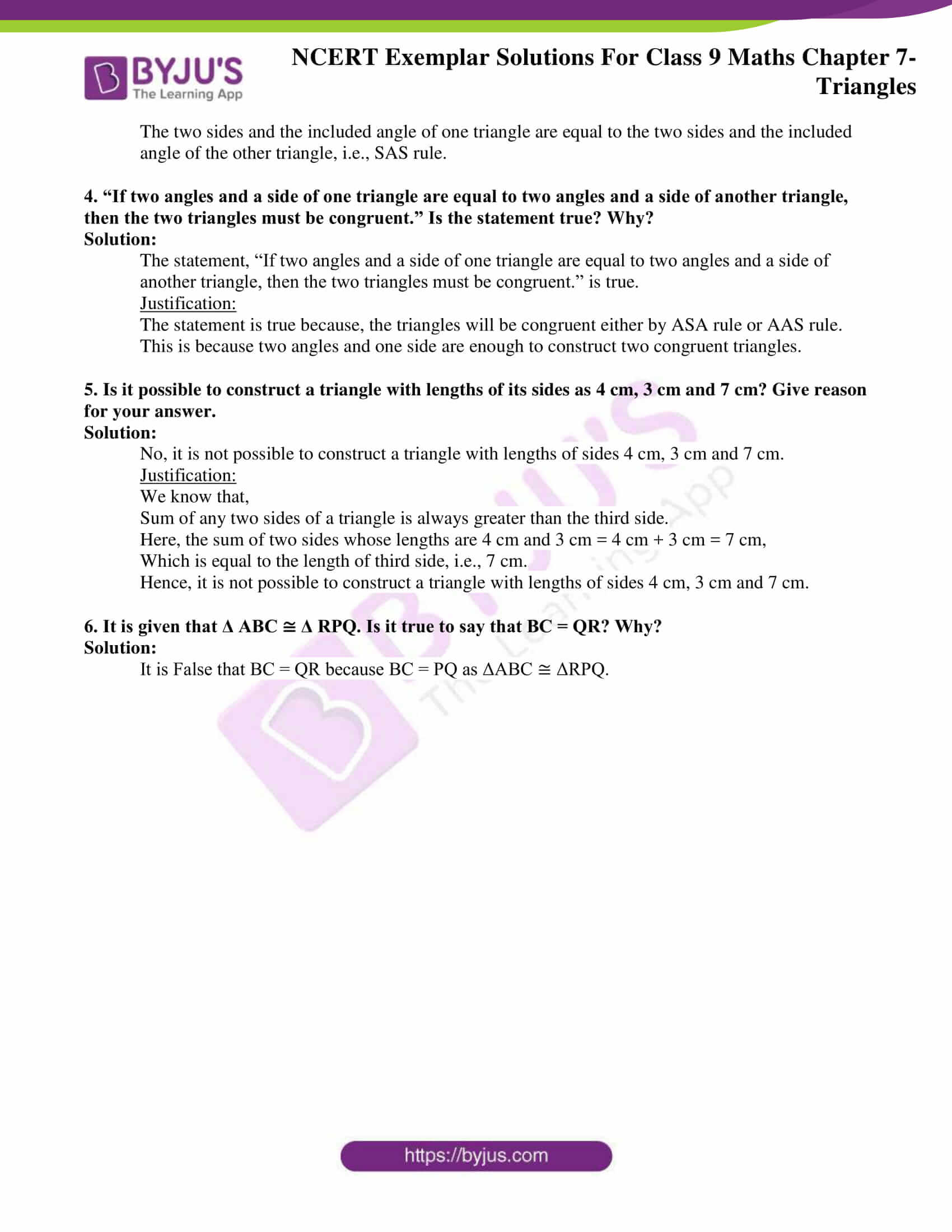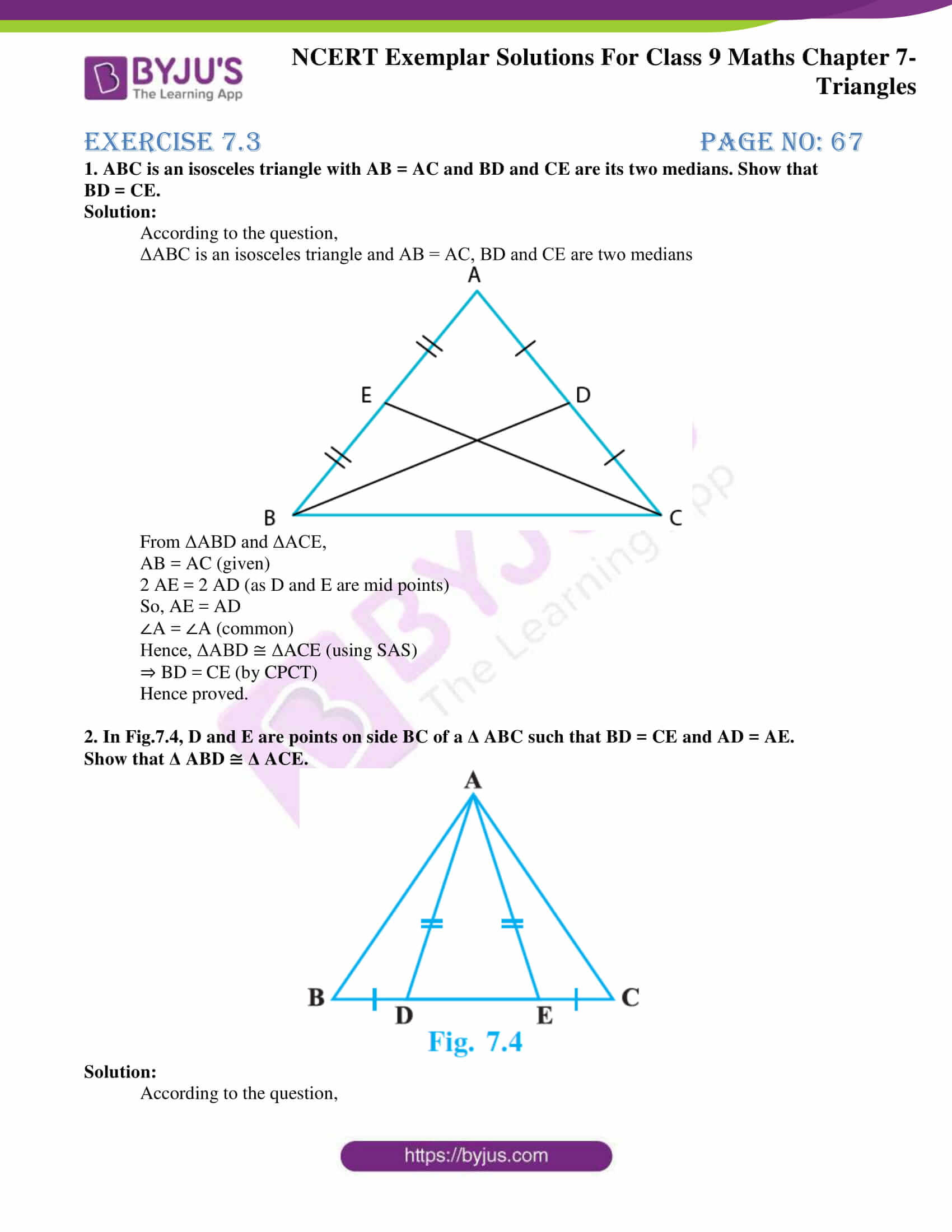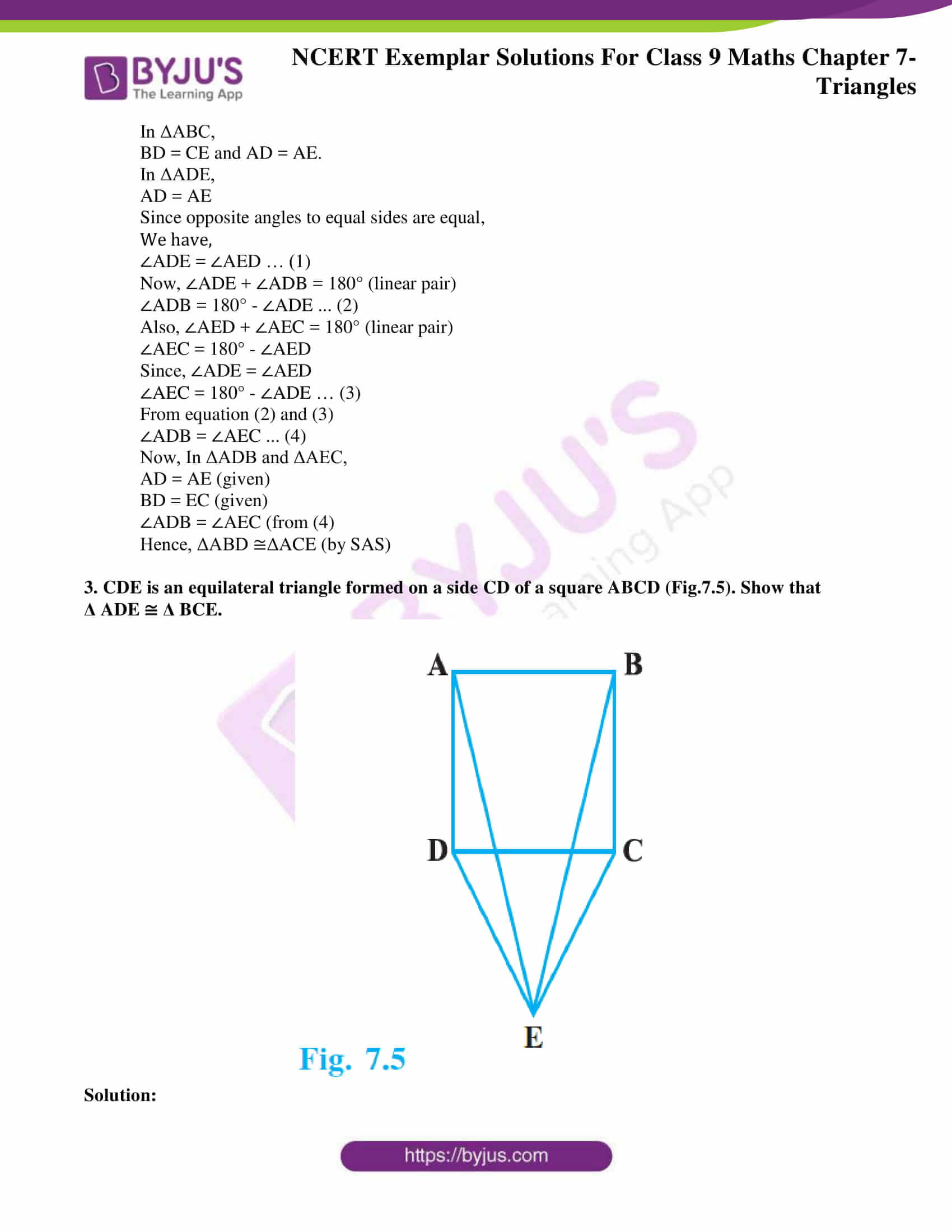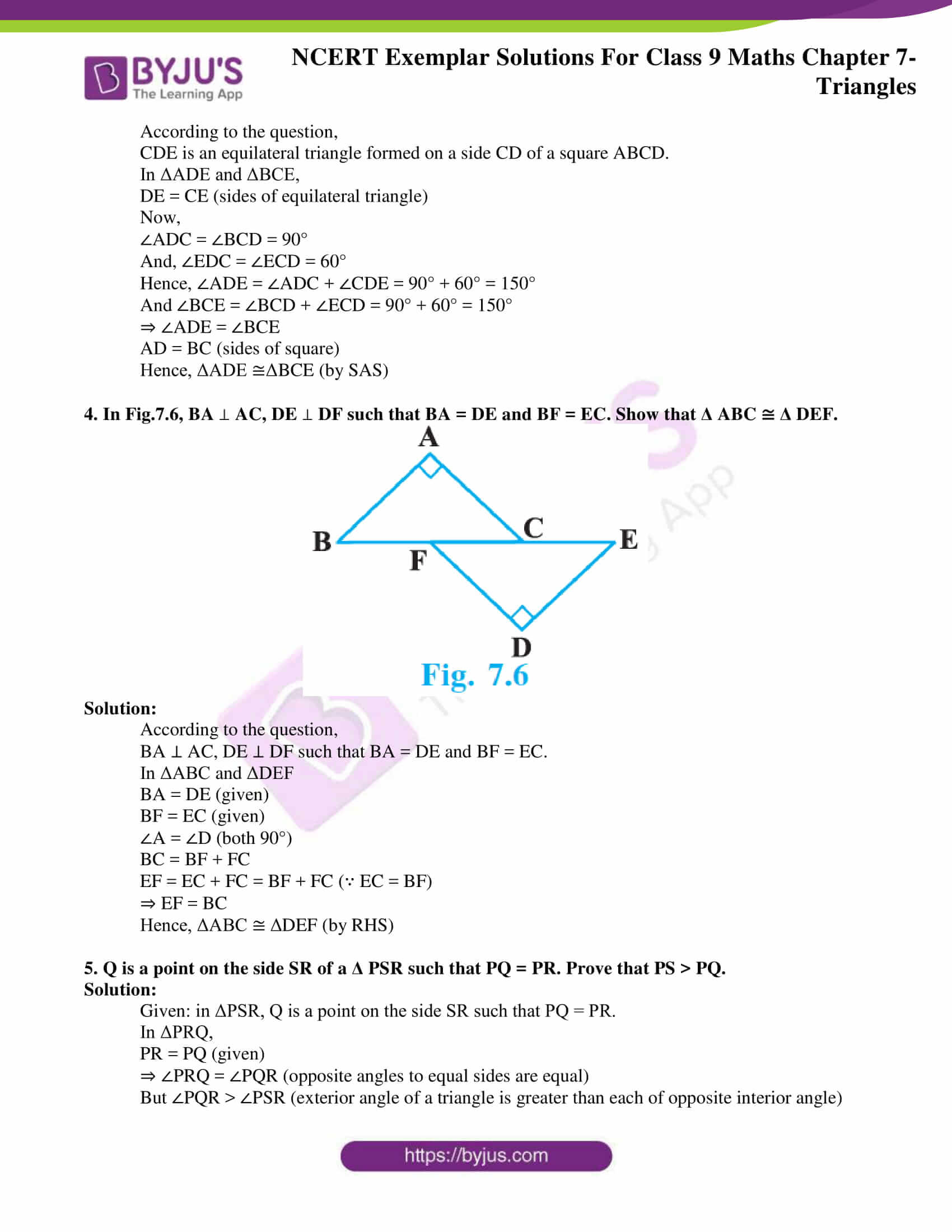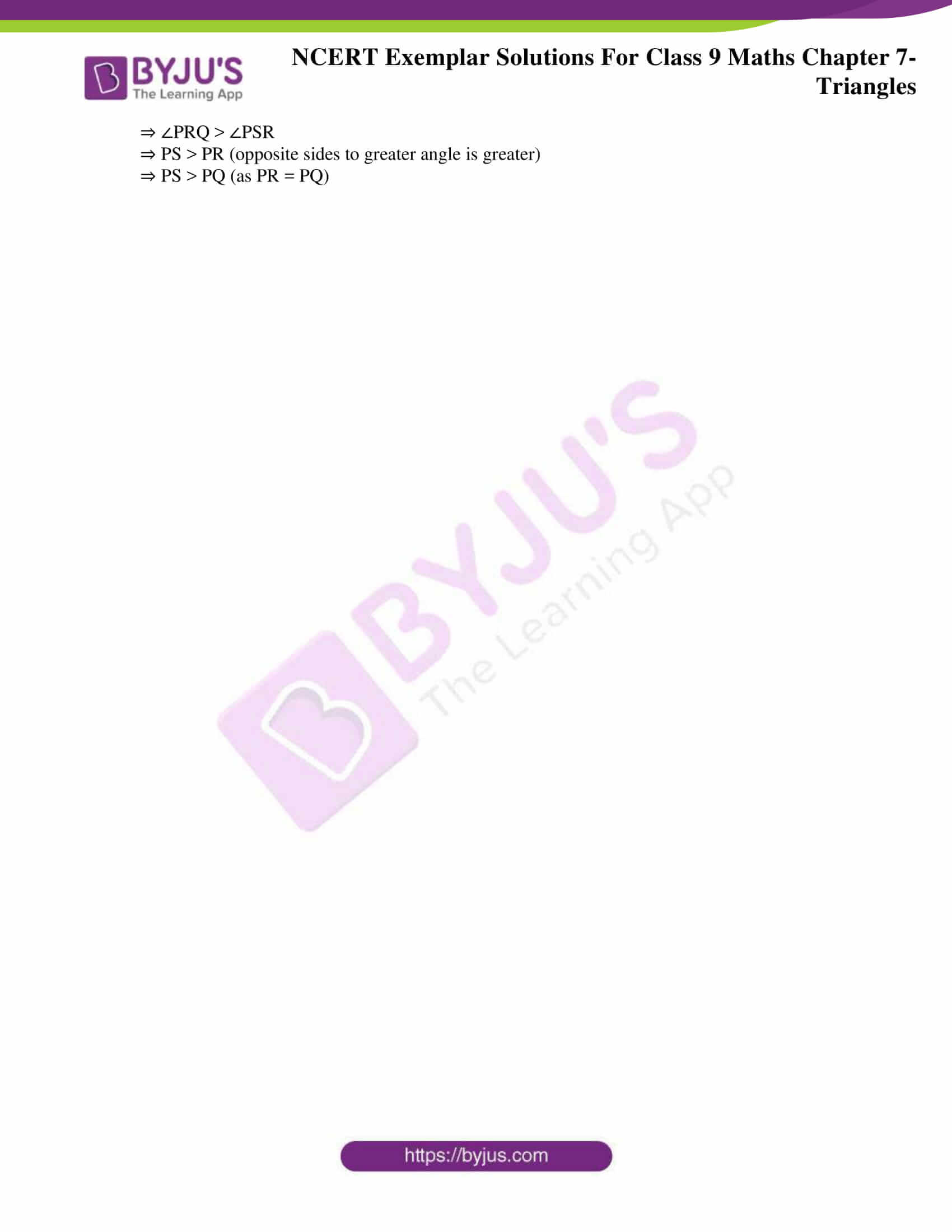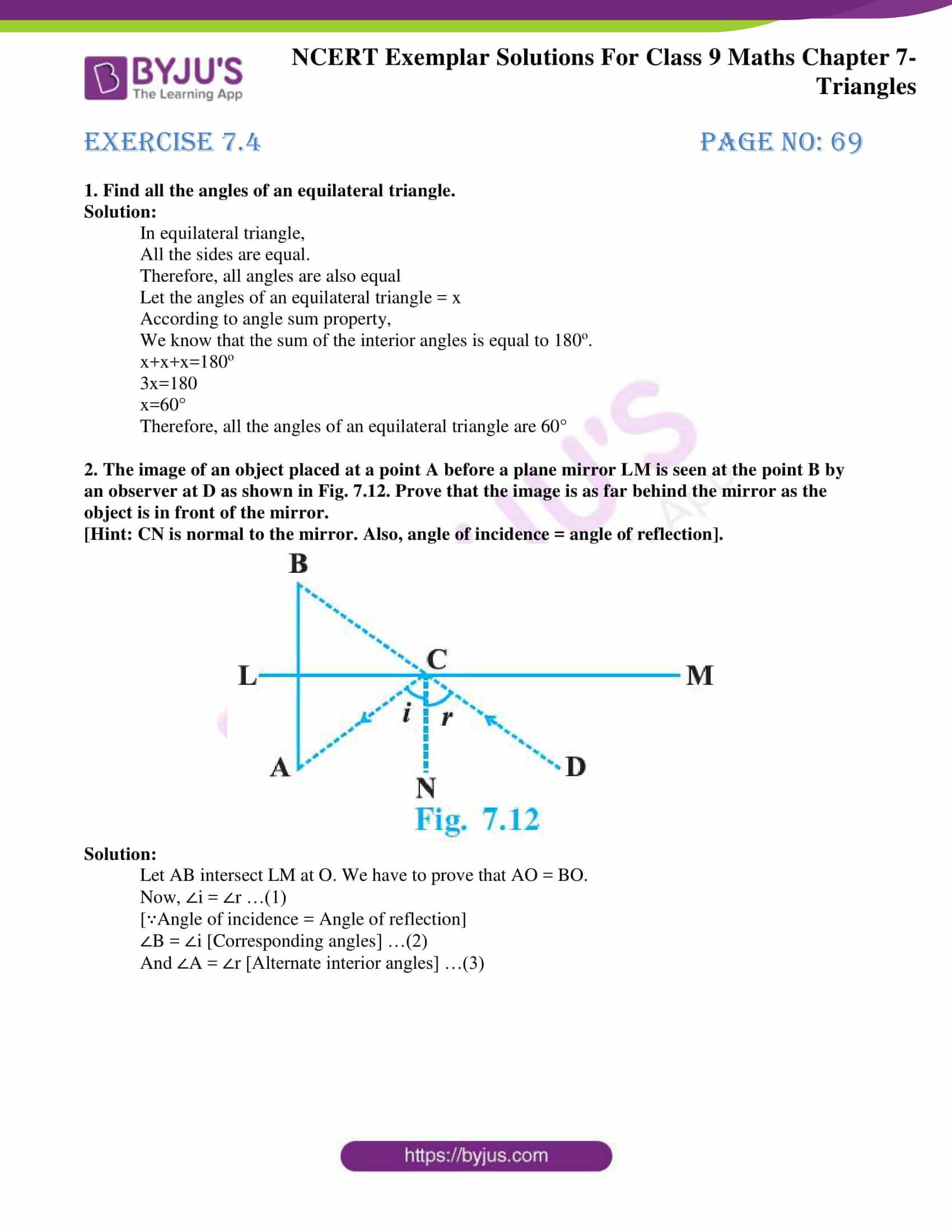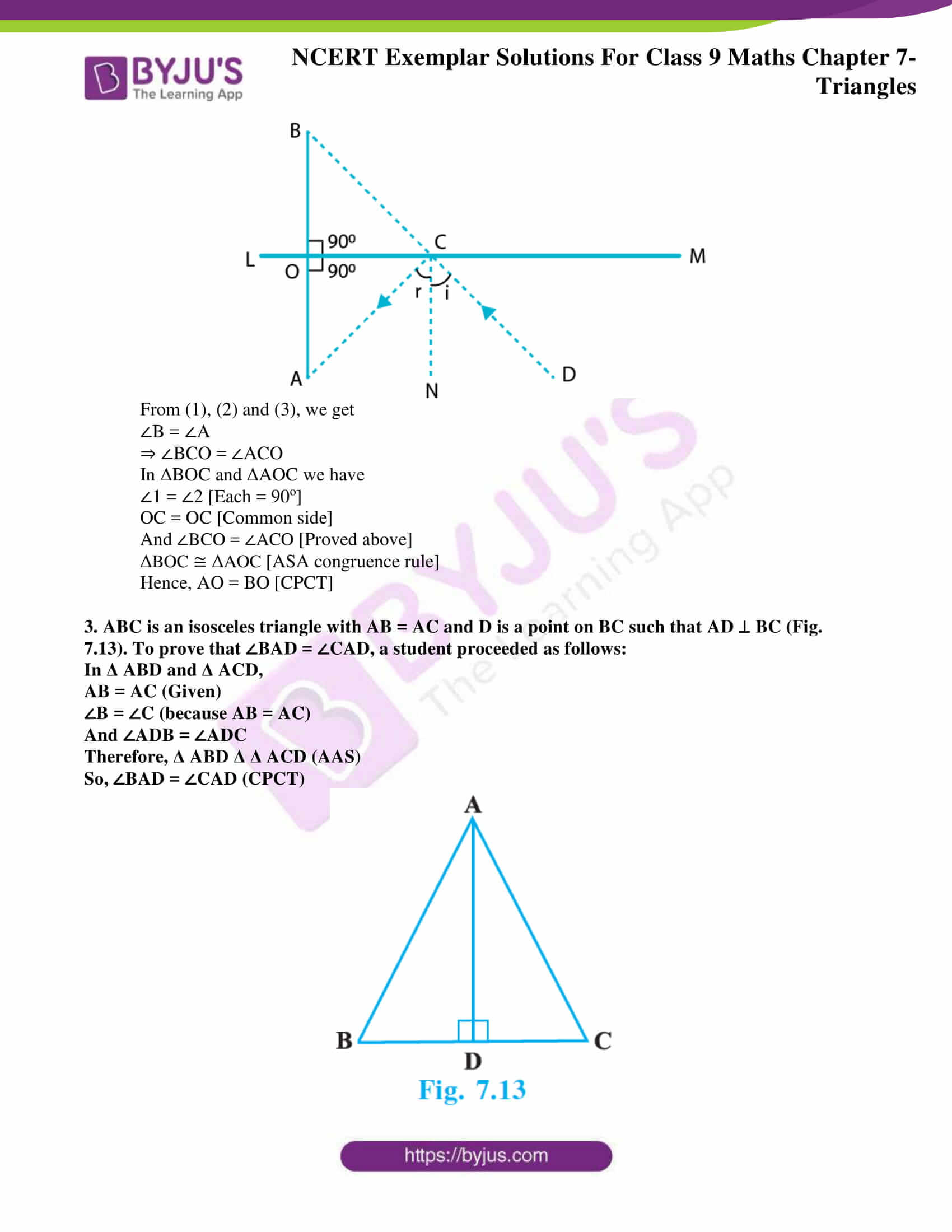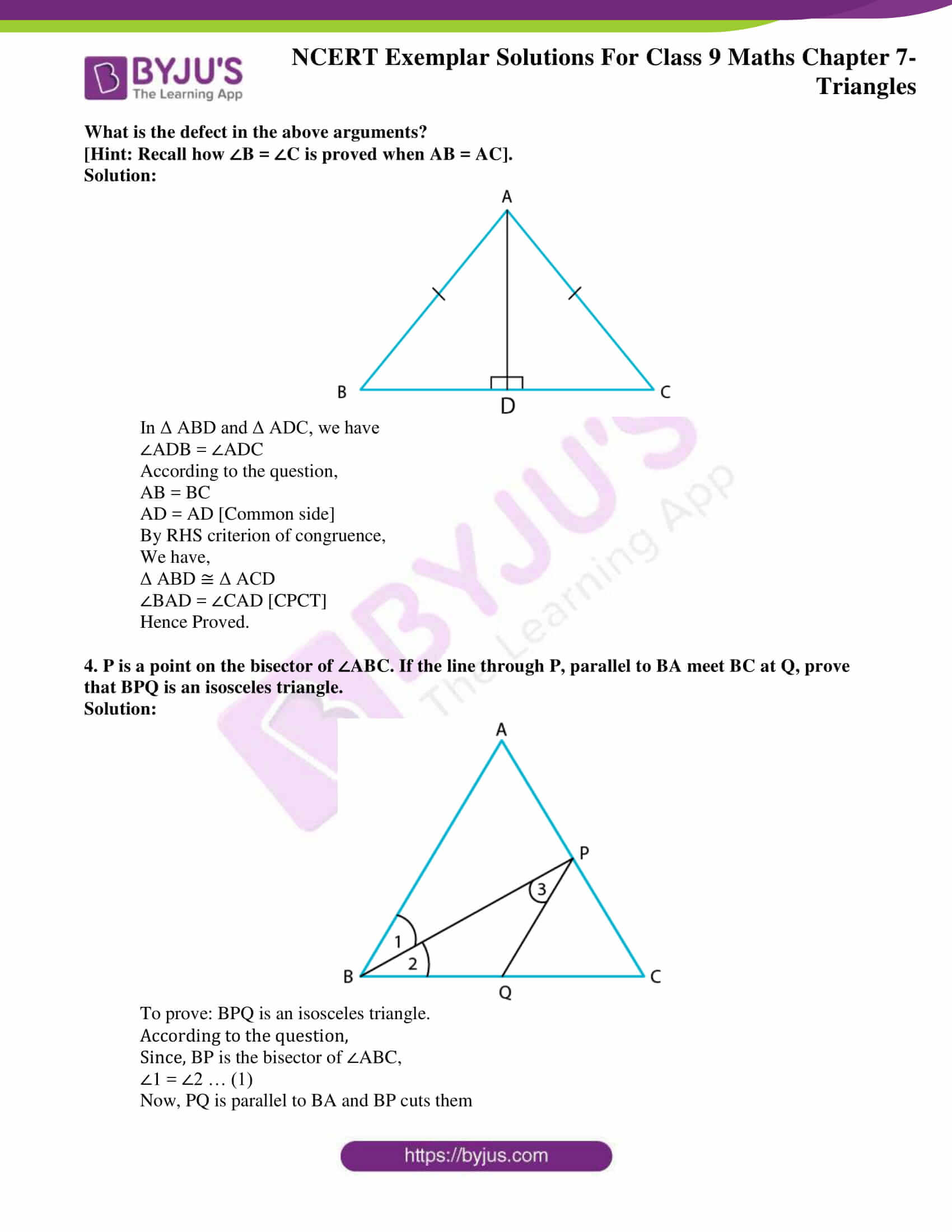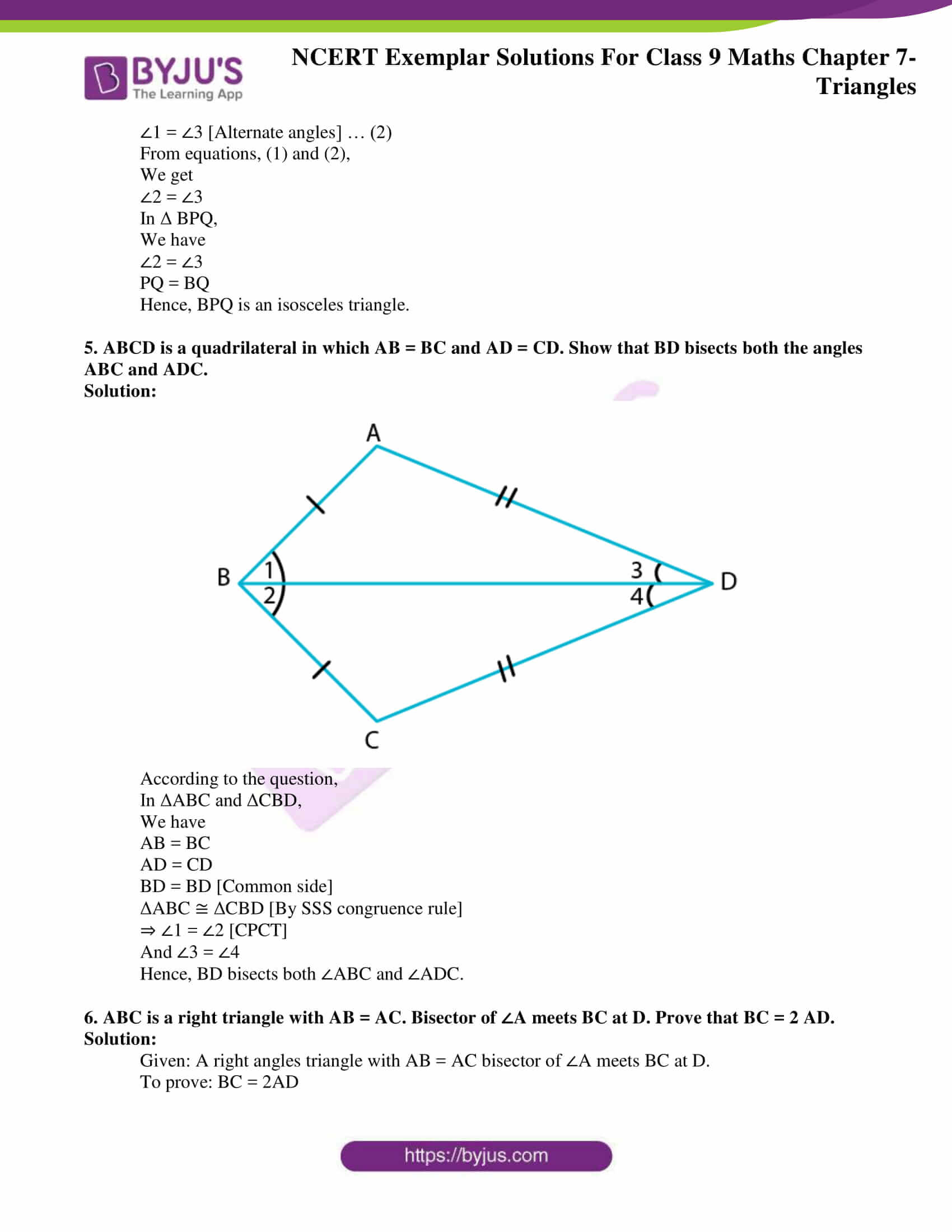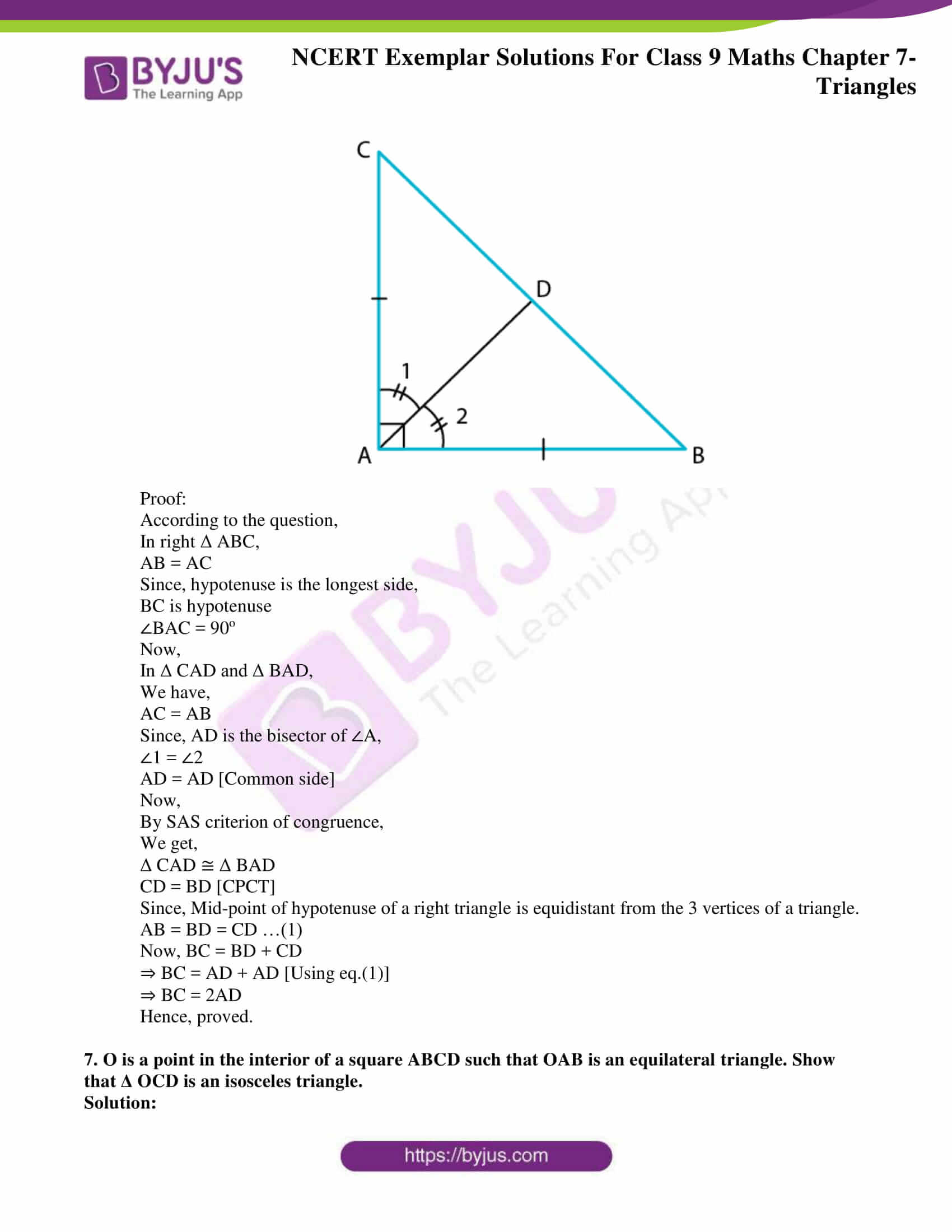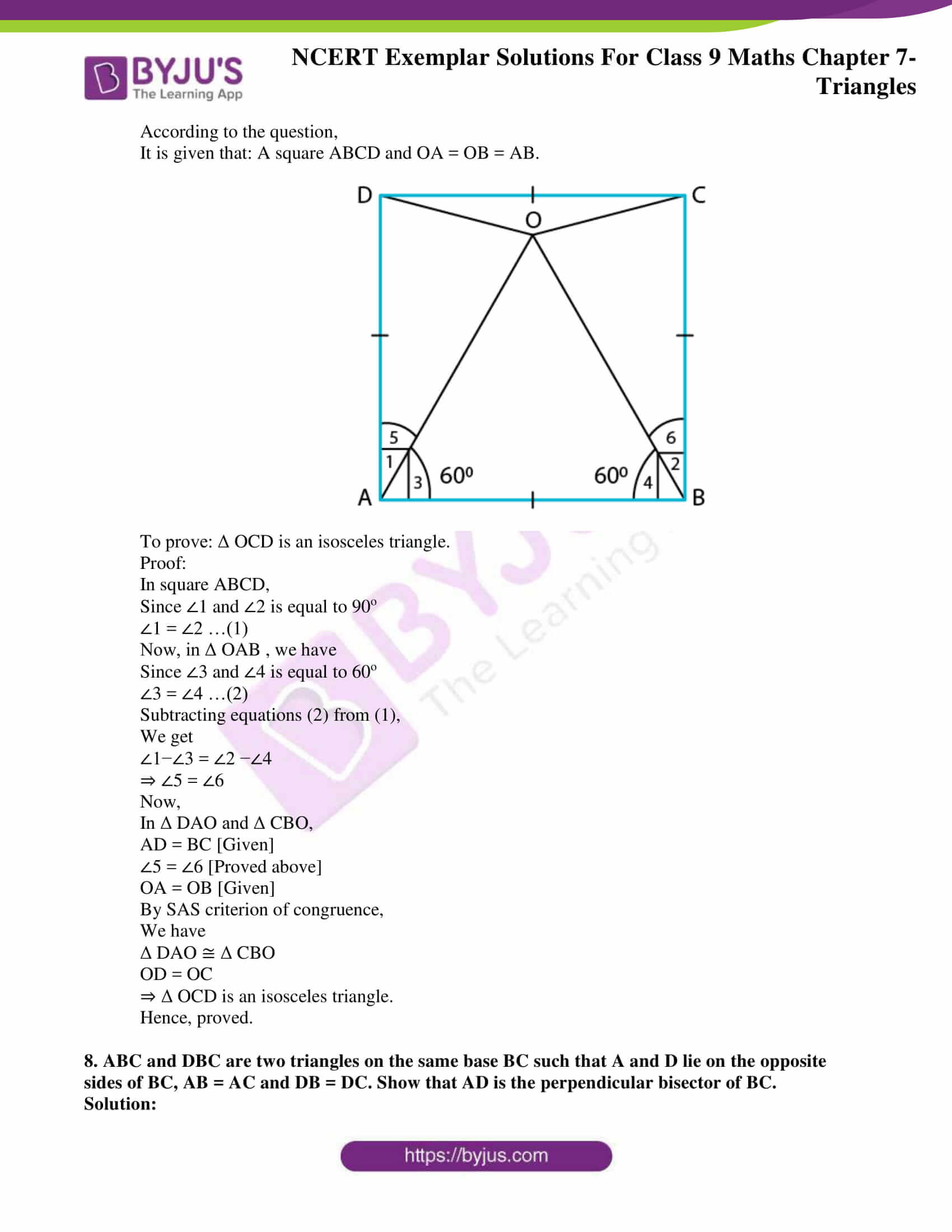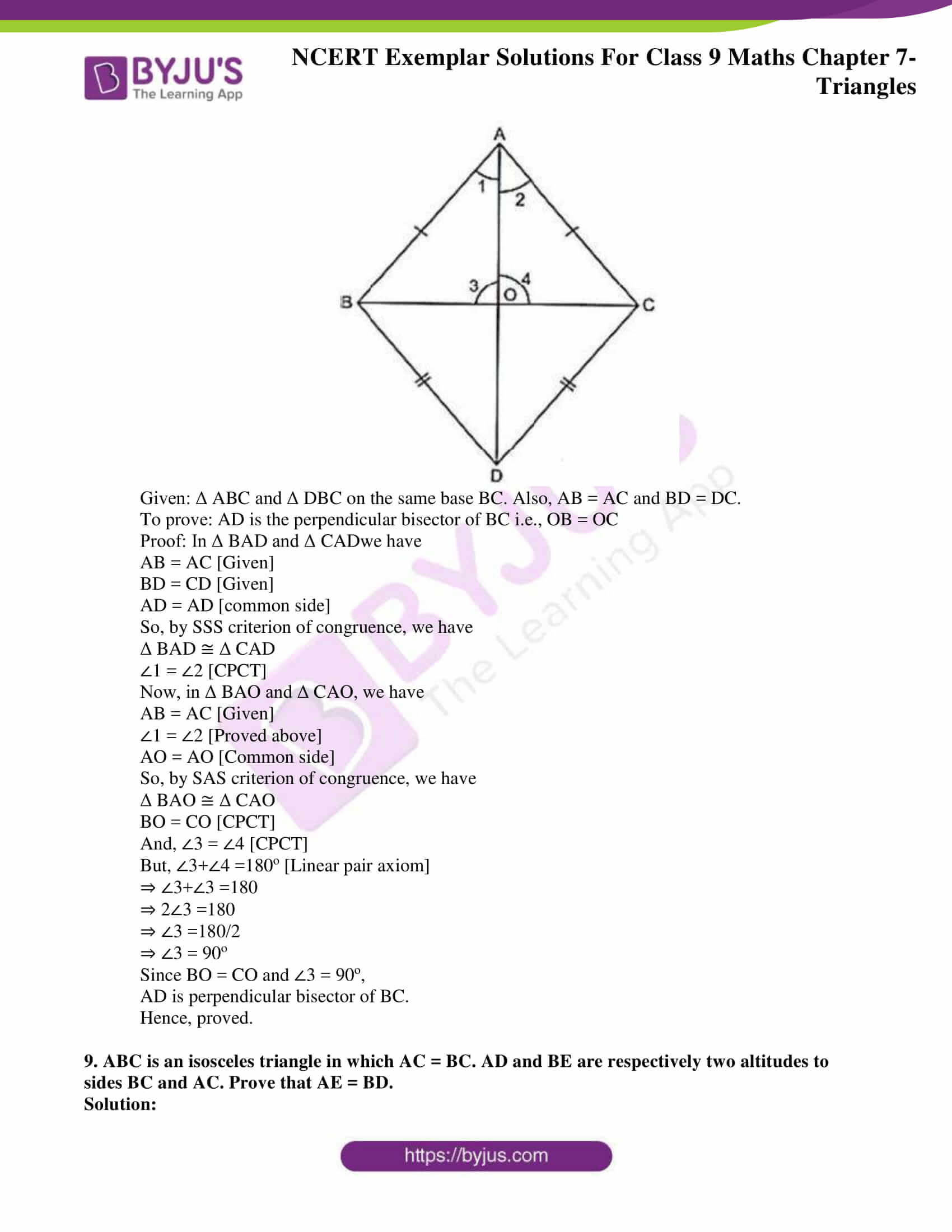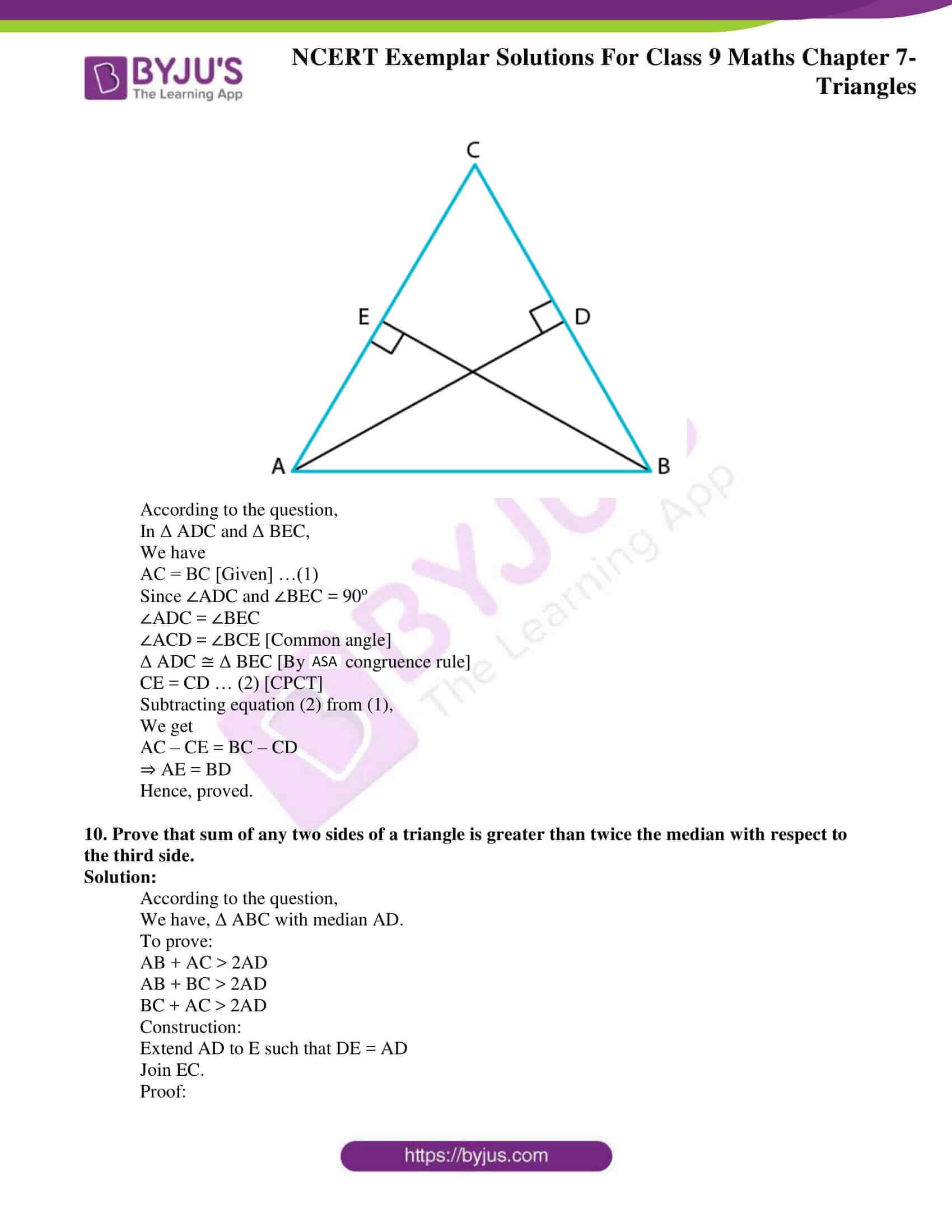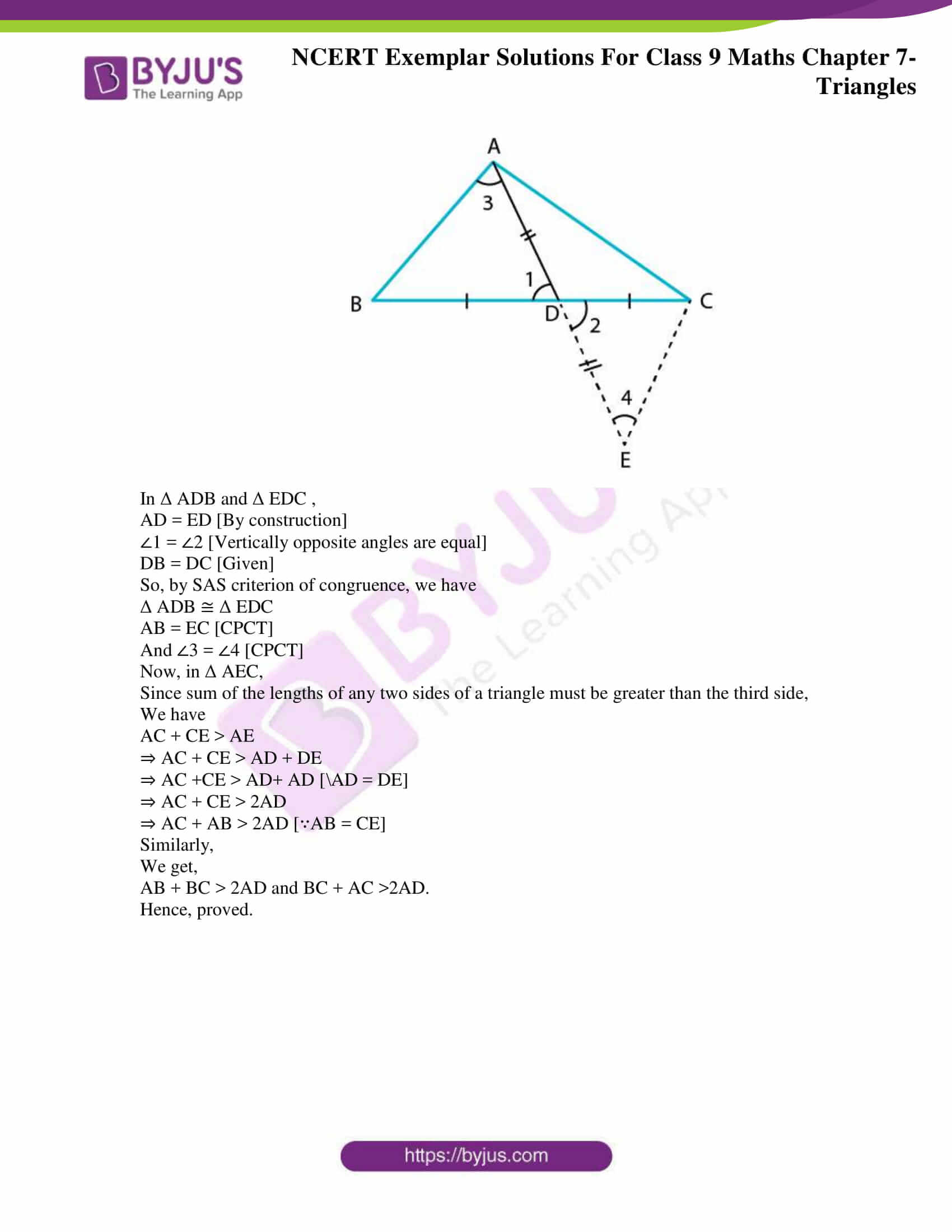### Access answers to NCERT Exemplar Solutions for Class 9 Maths Chapter 7 Triangles

Exercise 7.1 Page No: 64

In each of the following, write the correct answer:

1. Which of the following is not a criterion for congruence of triangles?

(A) SAS

(B) ASA

(C) SSA

(D) SSS

Solution:

(C) SSA

Explanation:

We know that,

Two triangles are congruent, if the side(S) and angles (A) of one triangle is equal to another.

And criterion for congruence of triangle are SAS, ASA, SSS, and RHS.

SSA is not the criterion for congruency of a triangle.

Hence, option C is the correct answer.

2. If AB = QR, BC = PR and CA = PQ, then

(A) Δ ABC ≅ ΔPQR

(B) ΔCBA ≅ ΔPRQ

(C) ΔBAC ≅ Δ RPQ

(D) Δ PQR ≅ Δ BCA

Solution:

(B) ΔCBA ≅ ΔPRQ

Explanation:

According to the question,

AB = QR, BC = PR and CA = PQ

Since, AB = QR, BC = PR and CA = PQ

We can say that,

A corresponds to Q, B corresponds to R, C corresponds to P.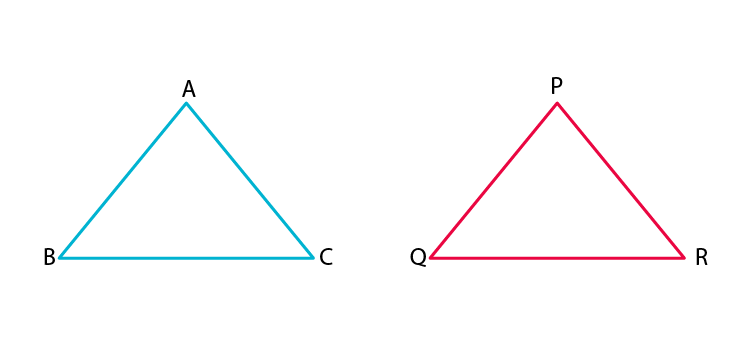Hence, (B) ΔCBA ≅ ΔPRQ

Hence, option B is the correct answer.

3. In Δ ABC, AB = AC and ∠B = 50°. Then ∠C is equal to

(A) 40° (B) 50° (C) 80° (D) 130°

Solution:

(B) 50°

Explanation:

According to the question,

Δ ABC, AB = AC and ∠B = 50°.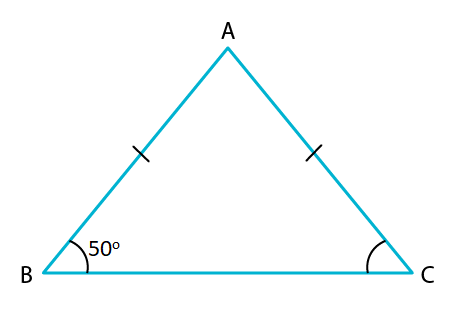Since, AB = AC

Δ ABC is an isosceles triangle.

Hence, ∠B = ∠C

∠B = 50° (given)

⇒ ∠C = 50°

Hence, option B is the correct option.

4. In Δ ABC, BC = AB and ∠B = 80°. Then ∠A is equal to

(A) 80° (B) 40° (C) 50° (D) 100°

Solution:

(C) 50°

Explanation:

Given: Δ ABC, BC = AB and ∠B = 80°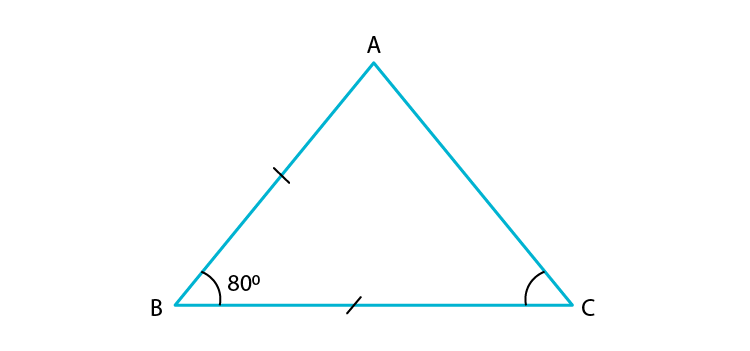Since, BC = AB

Δ ABC is an isosceles triangle.

Let, ∠C = ∠A = x

∠B = 80° (given)

We know that,

Using angle sum property,

Sum of interior angles of a triangle should be = 180o

∠A + ∠B + ∠C = 180°

⇒ x + 80° + x = 180°

⇒ 2x = 180° – 80°

⇒ 2x = 100°

⇒ x = 50°

Therefore, ∠C = ∠A = 50°

Hence, option C is the correct answer.

5. In Δ PQR, ∠R = ∠P and QR = 4 cm and PR = 5 cm. Then the length of PQ is

(A) 4 cm (B) 5 cm (C) 2 cm (D) 2.5 cm

Solution:

Given: ΔPQR, ∠R = ∠P and QR = 4 cm and PR = 5 cm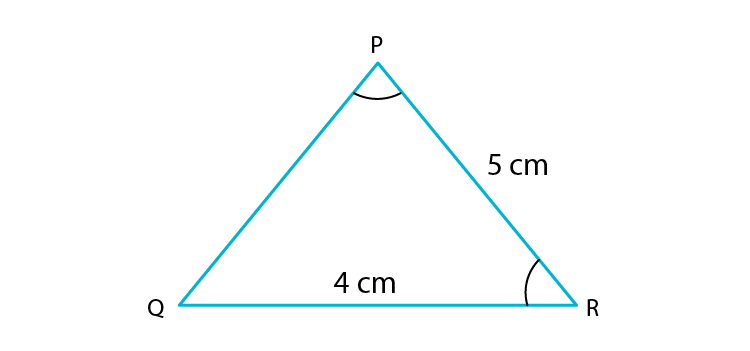Since, ∠R = ∠P

Δ PQR is an isosceles triangle.

Hence, PQ = QR

⇒ PQ = 4cm

Hence, option A is the correct answer.

Exercise 7.2 Page No: 65

1. In triangles ABC and PQR, ∠A = ∠Q and ∠B = ∠R. Which side of Δ PQR should be equal to side AB of Δ ABC so that the two triangles are congruent? Give reason for your answer.

Solution: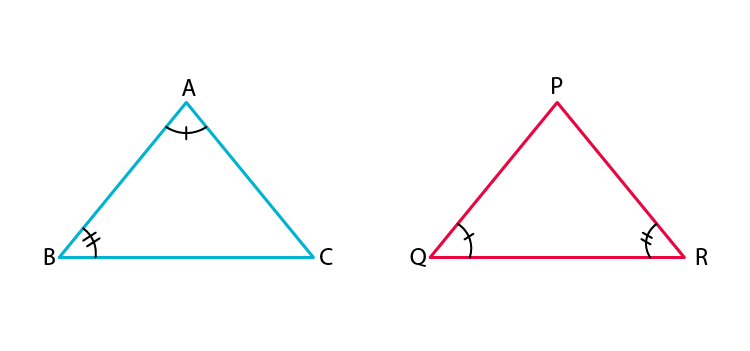In triangle ABC and PQR, we have

∠A = ∠Q [Given]

∠B = ∠R [Given]

For the triangle to be congruent, AB should be equal to QR.

Hence, triangle ABC and PQR can be congruent by ASA congruence rule.

2. In triangles ABC and PQR, ∠A = ∠Q and ∠B = ∠R. Which side of Δ PQR should be equal to side BC of Δ ABC so that the two triangles are congruent? Give reason for your answer.

Solution:In triangle ABC and PQR, we have

A = ∠Q and ∠B = ∠R [Given]

For the triangles to be congruent, we must have

BC = RP

Hence, triangle ABC and PQR will be congruent by AAS congruence rule.

3. “If two sides and an angle of one triangle are equal to two sides and an angle of another triangle, then the two triangles must be congruent.” Is the statement true? Why?

Solution:

No, the statement, “if two sides and an angle of one triangle are equal to two sides and an angle of another triangle, then the two triangles must be congruent” is false.

Justification:

Because by the congruent rule,

The two sides and the included angle of one triangle are equal to the two sides and the included angle of the other triangle, i.e., SAS rule.

4. “If two angles and a side of one triangle are equal to two angles and a side of another triangle, then the two triangles must be congruent.” Is the statement true? Why?

Solution:

The statement, “If two angles and a side of one triangle are equal to two angles and a side of another triangle, then the two triangles must be congruent.” is true.

Justification:

The statement is true because, the triangles will be congruent either by ASA rule or AAS rule. This is because two angles and one side are enough to construct two congruent triangles.

5. Is it possible to construct a triangle with lengths of its sides as 4 cm, 3 cm and 7 cm? Give reason for your answer.

Solution:

No, it is not possible to construct a triangle with lengths of sides 4 cm, 3 cm and 7 cm.

Justification:

We know that,

Sum of any two sides of a triangle is always greater than the third side.

Here, the sum of two sides whose lengths are 4 cm and 3 cm = 4 cm + 3 cm = 7 cm,

Which is equal to the length of third side, i.e., 7 cm.

Hence, it is not possible to construct a triangle with lengths of sides 4 cm, 3 cm and 7 cm.

6. It is given that Δ ABC ≅ Δ RPQ. Is it true to say that BC = QR? Why?

Solution:

It is False that BC = QR because BC = PQ as ΔABC ≅ ΔRPQ.

Exercise 7.3 Page No: 67

1. ABC is an isosceles triangle with AB = AC and BD and CE are its two medians. Show that

BD = CE.

Solution:

According to the question,

ΔABC is an isosceles triangle and AB = AC, BD and CE are two medians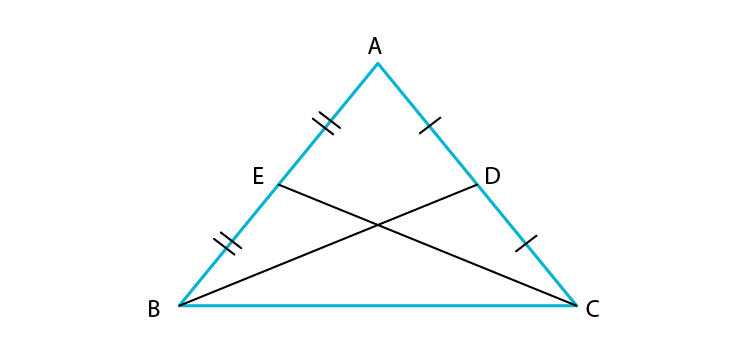From ΔABD and ΔACE,

AB = AC (given)

2 AE = 2 AD (as D and E are mid points)

∠A = ∠A (common)

Hence, ΔABD ≅ ΔACE (using SAS)

⇒ BD = CE (by CPCT)

Hence proved.

2. In Fig.7.4, D and E are points on side BC of a Δ ABC such that BD = CE and AD = AE.

Show that Δ ABD ≅ Δ ACE.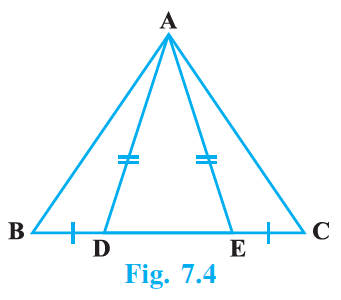Solution:

According to the question,

In ΔABC,

BD = CE and AD = AE.

Since opposite angles to equal sides are equal,

We have,

Also, ∠AED + ∠AEC = 180° (linear pair)

∠AEC = 180° – ∠AED

∠AEC = 180° – ∠ADE … (3)

From equation (2) and (3)

BD = EC (given)

Hence, ΔABD ≅ΔACE (by SAS)

3. CDE is an equilateral triangle formed on a side CD of a square ABCD (Fig.7.5). Show that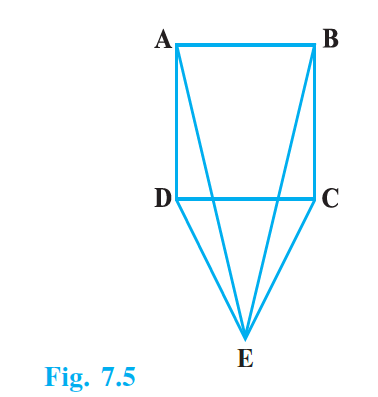Solution:

According to the question,

CDE is an equilateral triangle formed on a side CD of a square ABCD.

DE = CE (sides of equilateral triangle)

Now,

And, ∠EDC = ∠ECD = 60°

Hence, ∠ADE = ∠ADC + ∠CDE = 90° + 60° = 150°

And ∠BCE = ∠BCD + ∠ECD = 90° + 60° = 150°

AD = BC (sides of square)

4. In Fig.7.6, BA AC, DE DF such that BA = DE and BF = EC. Show that Δ ABC ≅ Δ DEF.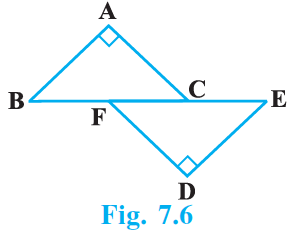Solution:

According to the question,

BA ⊥ AC, DE ⊥ DF such that BA = DE and BF = EC.

In ΔABC and ΔDEF

BA = DE (given)

BF = EC (given)

∠A = ∠D (both 90°)

BC = BF + FC

EF = EC + FC = BF + FC (∵ EC = BF)

⇒ EF = BC

Hence, ΔABC ≅ ΔDEF (by RHS)

5. Q is a point on the side SR of a Δ PSR such that PQ = PR. Prove that PS > PQ.

Solution:

Given: in ΔPSR, Q is a point on the side SR such that PQ = PR.

In ΔPRQ,

PR = PQ (given)

⇒ ∠PRQ = ∠PQR (opposite angles to equal sides are equal)

But ∠PQR > ∠PSR (exterior angle of a triangle is greater than each of opposite interior angle)

⇒ ∠PRQ > ∠PSR

⇒ PS > PR (opposite sides to greater angle is greater)

⇒ PS > PQ (as PR = PQ)

Exercise 7.4 Page No: 69

1. Find all the angles of an equilateral triangle.

Solution:

In equilateral triangle,

All the sides are equal.

Therefore, all angles are also equal

Let the angles of an equilateral triangle = x

According to angle sum property,

We know that the sum of the interior angles is equal to 180o.

x+x+x=180o

3x=180

x=60°

Therefore, all the angles of an equilateral triangle are 60°

2. The image of an object placed at a point A before a plane mirror LM is seen at the point B by an observer at D as shown in Fig. 7.12. Prove that the image is as far behind the mirror as the object is in front of the mirror.

[Hint: CN is normal to the mirror. Also, angle of incidence = angle of reflection].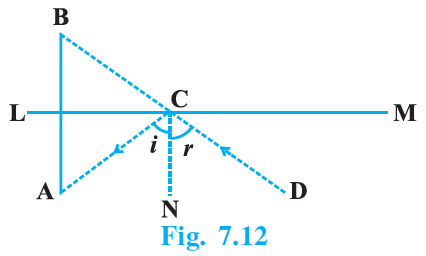Solution:

Let AB intersect LM at O. We have to prove that AO = BO.

Now, ∠i = ∠r …(1)

[∵Angle of incidence = Angle of reflection]

∠B = ∠i [Corresponding angles] …(2)

And ∠A = ∠r [Alternate interior angles] …(3)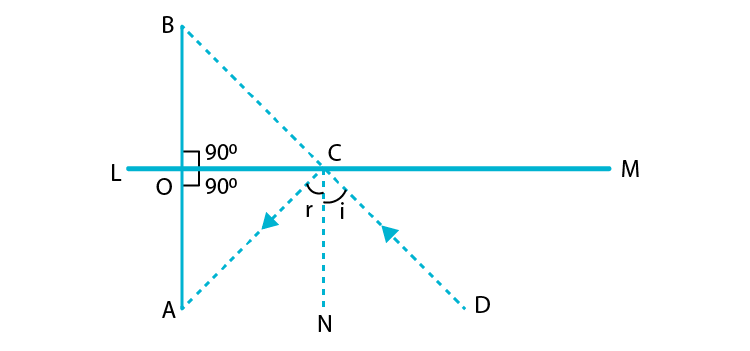From (1), (2) and (3), we get

∠B = ∠A

⇒ ∠BCO = ∠ACO

In ΔBOC and ΔAOC we have

∠1 = ∠2 [Each = 90o]

OC = OC [Common side]

And ∠BCO = ∠ACO [Proved above]

ΔBOC ≅ ΔAOC [ASA congruence rule]

Hence, AO = BO [CPCT]

3. ABC is an isosceles triangle with AB = AC and D is a point on BC such that AD ⊥ BC (Fig. 7.13). To prove that ∠BAD = ∠CAD, a student proceeded as follows:

In Δ ABD and Δ ACD,

AB = AC (Given)

∠B = ∠C (because AB = AC)

Therefore, Δ ABD Δ Δ ACD (AAS)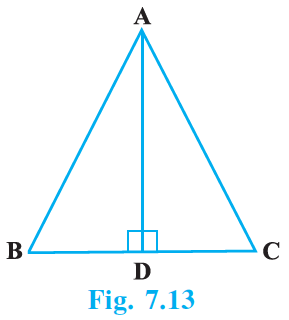What is the defect in the above arguments?

[Hint: Recall how ∠B = ∠C is proved when AB = AC].

Solution: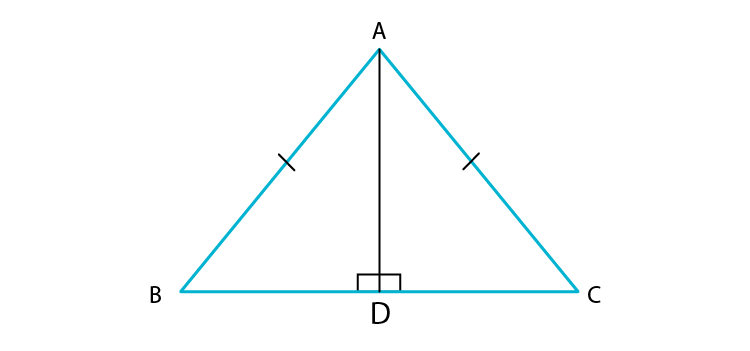In Δ ABD and Δ ADC, we have

According to the question,

AB = BC

By RHS criterion of congruence,

We have,

Δ ABD ≅ Δ ACD

Hence Proved.

4. P is a point on the bisector of ∠ABC. If the line through P, parallel to BA meet BC at Q, prove that BPQ is an isosceles triangle.

Solution: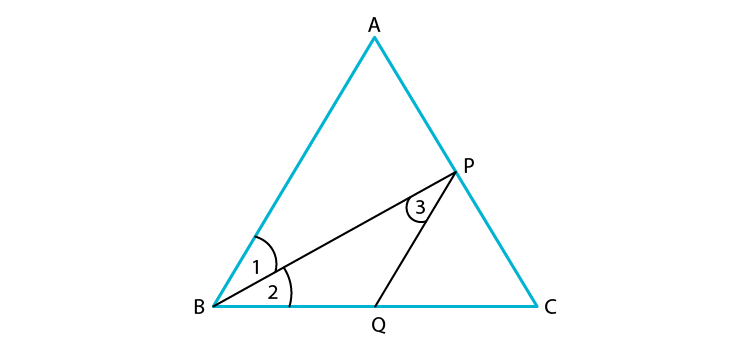To prove: BPQ is an isosceles triangle.

According to the question,

Since, BP is the bisector of ∠ABC,

∠1 = ∠2 … (1)

Now, PQ is parallel to BA and BP cuts them

∠1 = ∠3 [Alternate angles] … (2)

From equations, (1) and (2),

We get

∠2 = ∠3

In Δ BPQ,

We have

∠2 = ∠3

PQ = BQ

Hence, BPQ is an isosceles triangle.

5. ABCD is a quadrilateral in which AB = BC and AD = CD. Show that BD bisects both the angles ABC and ADC.

Solution: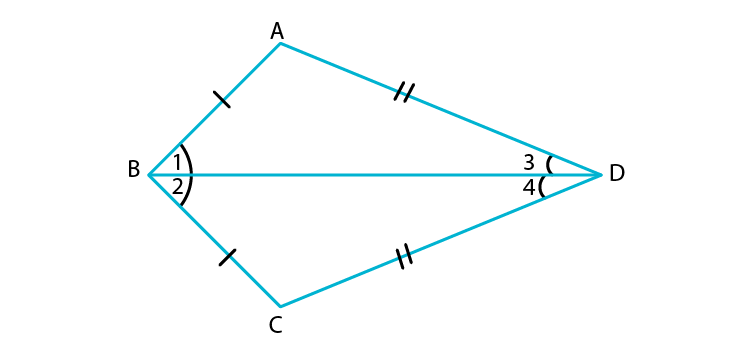According to the question,

In ΔABC and ΔCBD,

We have

AB = BC

BD = BD [Common side]

ΔABC ≅ ΔCBD [By SSS congruence rule]

⇒ ∠1 = ∠2 [CPCT]

And ∠3 = ∠4

Hence, BD bisects both ∠ABC and ∠ADC.

6. ABC is a right triangle with AB = AC. Bisector of ∠A meets BC at D. Prove that BC = 2 AD.

Solution:

Given: A right angles triangle with AB = AC bisector of ∠A meets BC at D.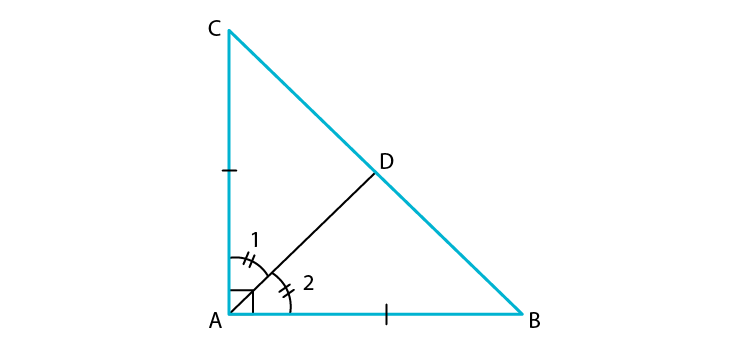Proof:

According to the question,

In right Δ ABC,

AB = AC

Since, hypotenuse is the longest side,

BC is hypotenuse

∠BAC = 90o

Now,

We have,

AC = AB

Since, AD is the bisector of ∠A,

∠1 = ∠2

Now,

By SAS criterion of congruence,

We get,

CD = BD [CPCT]

Since, Mid-point of hypotenuse of a right triangle is equidistant from the 3 vertices of a triangle.

AB = BD = CD …(1)

Now, BC = BD + CD

Hence, proved.

7. O is a point in the interior of a square ABCD such that OAB is an equilateral triangle. Show that Δ OCD is an isosceles triangle.

Solution:

According to the question,

It is given that: A square ABCD and OA = OB = AB.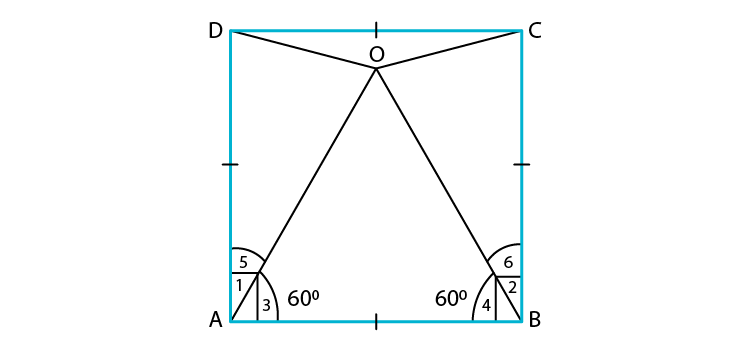To prove: Δ OCD is an isosceles triangle.

Proof:

In square ABCD,

Since ∠1 and ∠2 is equal to 90o

∠1 = ∠2 …(1)

Now, in Δ OAB , we have

Since ∠3 and ∠4 is equal to 60o

∠3 = ∠4 …(2)

Subtracting equations (2) from (1),

We get

∠1−∠3 = ∠2 −∠4

⇒ ∠5 = ∠6

Now,

In Δ DAO and Δ CBO,

∠5 = ∠6 [Proved above]

OA = OB [Given]

By SAS criterion of congruence,

We have

Δ DAO ≅ Δ CBO

OD = OC

⇒ Δ OCD is an isosceles triangle.

Hence, proved.

8. ABC and DBC are two triangles on the same base BC such that A and D lie on the opposite sides of BC, AB = AC and DB = DC. Show that AD is the perpendicular bisector of BC.

Solution: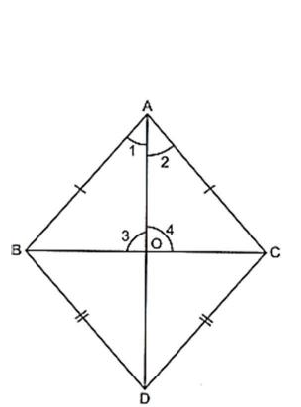Given: Δ ABC and Δ DBC on the same base BC. Also, AB = AC and BD = DC.

To prove: AD is the perpendicular bisector of BC i.e., OB = OC

AB = AC [Given]

BD = CD [Given]

So, by SSS criterion of congruence, we have

∠1 = ∠2 [CPCT]

Now, in Δ BAO and Δ CAO, we have

AB = AC [Given]

∠1 = ∠2 [Proved above]

AO = AO [Common side]

So, by SAS criterion of congruence, we have

Δ BAO ≅ Δ CAO

BO = CO [CPCT]

And, ∠3 = ∠4 [CPCT]

But, ∠3+∠4 =180o [Linear pair axiom]

⇒ ∠3+∠3 =180

⇒ 2∠3 =180

⇒ ∠3 =180/2

⇒ ∠3 = 90o

Since BO = CO and ∠3 = 90o,

AD is perpendicular bisector of BC.

Hence, proved.

9. ABC is an isosceles triangle in which AC = BC. AD and BE are respectively two altitudes to sides BC and AC. Prove that AE = BD.

Solution: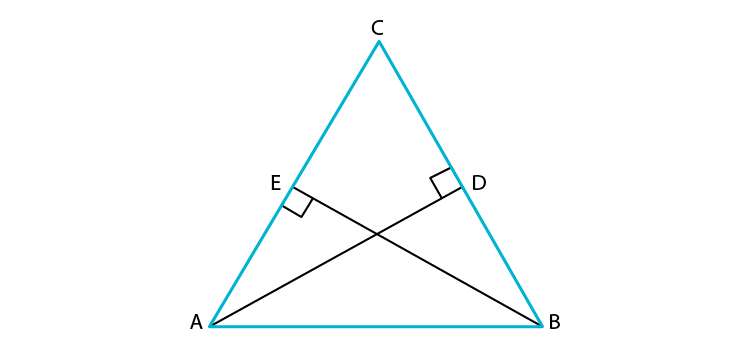According to the question,

In Δ ADC and Δ BEC,

We have

AC = BC [Given] …(1)

Since ∠ADC and ∠BEC = 90o

∠ACD = ∠BCE [Common angle]

Δ ADC ≅ Δ BEC [By ASA congruence rule]

CE = CD … (2) [CPCT]

Subtracting equation (2) from (1),

We get

AC – CE = BC – CD

⇒ AE = BD

Hence, proved.

10. Prove that sum of any two sides of a triangle is greater than twice the median with respect to the third side.

Solution:

According to the question,

We have, Δ ABC with median AD.

To prove:

Construction:

Join EC.

Proof: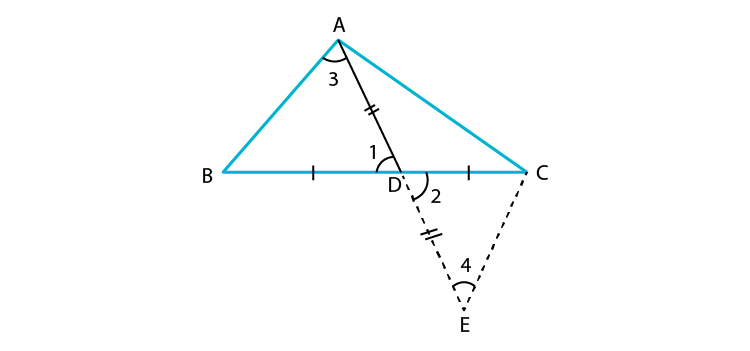In Δ ADB and Δ EDC ,

∠1 = ∠2 [Vertically opposite angles are equal]

DB = DC [Given]

So, by SAS criterion of congruence, we have

AB = EC [CPCT]

And ∠3 = ∠4 [CPCT]

Now, in Δ AEC,

Since sum of the lengths of any two sides of a triangle must be greater than the third side,

We have

AC + CE > AE

⇒ AC + CE > AD + DE

⇒ AC + CE > 2AD

⇒ AC + AB > 2AD [∵AB = CE]

Similarly,

We get,

Hence, proved.

Students are also suggested to use our study materials for Class 9, such as Exemplar books, notes, NCERT Solutions while preparing for exams and to have a quick review from it. Also, the previous year question papers and sample papers will give them the idea of questions asked from Chapter 7 Triangles.

Download BYJU’S-The learning app to get personalized videos explaining various concepts of Maths such as triangles and congruency and of related topics with the help of pictures and video contents. Also, get these exemplars for all the chapters of Class 9 Maths subject with us.

## Frequently Asked Questions on NCERT Exemplar Solutions for Class 9 Maths Chapter 7

### What are the crucial topics covered in the NCERT Exemplar Solutions for Class 9 Maths Chapter 7?

The crucial topics covered in the NCERT Exemplar Solutions for Class 9 Maths Chapter 7 are –
1.Congruent triangles
2. Criteria or conditions for congruence of triangles
3. Inequalities in a Triangle

### Discuss the fundamental concepts of congruence of triangles in the NCERT Exemplar Solutions for Class 9 Maths Chapter 7.

Two triangles are said to be congruent if all three corresponding sides are equal and all the three corresponding angles are equal in measure. These triangles can be slid, rotated, flipped and turned to look identical. If repositioned, they coincide with each other. The symbol of congruence is’ ≅’..

### Can I get the NCERT Exemplar Solutions for Class 9 Maths Chapter 7 in PDF format?

Yes, students can download the NCERT Exemplar Solutions for Class 9 Maths Chapter 7 in PDF format in BYJU’S. The solutions for the exercise wise problems are designed by the highly experienced faculty based on the latest syllabus of the CBSE board. Students can also cross check their answers with the solutions PDF in order to get a clear idea about the other methods of solving the complex problems effortlessly.